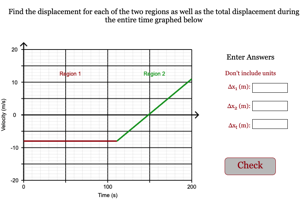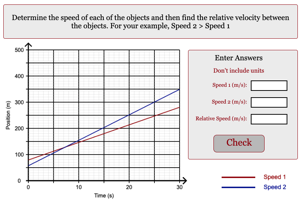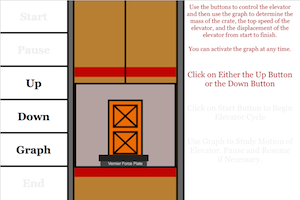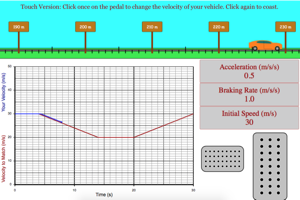Programs for 2nd Law (Graphical)
Below are all the programs that might be used with this topic. Click on "See Resources" to see if there are any student directions, lab sheets or other materials that have been created for this program.
Freestyle Graphing of Motion

LabConstant Velocity Graph

HomeworkFinding Distance From Velocity Graph Simple

HomeworkFinding Distance From Velocity Graph

HomeworkFinding Distance From Velocity Graph (w/ Negatives)

HomeworkRelative Velocity from Graph

HomeworkFinding Instantaneous Velocity from a P vs. T graph

HomeworkFinding Acceleration from a P vs. T graph

HomeworkForces on Elevator

HomeworkVelocity Graph Matching Challenge

Game